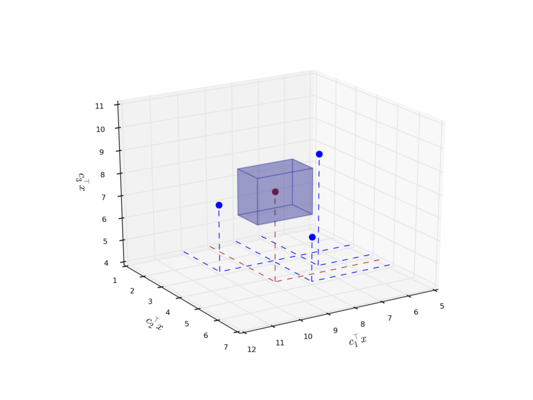Multi-objective optimization is concerned with optimizing several conflicting objectives at once.It can be considered as a generalization of single-objective optimization with numerous applications that range from health care, sustainable manufacturing, economics and social sciences to traffic and logistics. Roughly speaking, basically any real-world application that can be modeled as an optimization problem might be considered as a multi-criteria optimization problem if enough data is available. In contrast to the single-objective case, it is generally impossible to compute a single solution that optimizes all objectives simultaneously. Instead, we have to deal with trade-offs and distinguish between non-dominated points that cannot be improved upon (on at least one objective without getting worse at another) and dominated points that can be improved upon. In this project we pursue a new concept to partition the set of non-dominated points. This approach enables us to solve general integer programs by combining the research goals of achieving theoretical efficiency, of implementing practical algorithmic approaches and of being able to approximate the entire set of non-dominated points via a subset of non-dominated points with some kind of approximation guarantee. Furthermore, we aim at making all project results available to the optimization community via implementations to PolySCIP, our solver for multi-objective linear and integer programs.

### Description of the project

In this project we consider multi-objective integer programs which can be expressed as

$\min~ (c_1^\top x,\ldots, c_k^\top x)~\text{s.t.}~ Ax \geq b, x \in \mathbb{Z}^n$

where $k \geq 2$ is the number of given objectives. Given a multi-criteria integer program, our goal is to compute the entire set of non-dominated points.

#### A new concept for partitioning the set of non-dominated points

Our approach is based on the following new concept for non-dominated points: Let $y$ be a non-dominated point for the given multi-objective integer program with $k$ objectives. We define $y$ to be polynon-dominated if there is $i \in \{1,\ldots,k\}$ such that the projection of $y$ onto the $1,\ldots,i-1,i+1,\ldots,k$-coordinates is non-dominated for the given multi-objective integer program where the $i$-th objective is neglected. Otherwise, $y$ is called mononon-dominated.

We can show that the set of polynon-dominated points yields lower and upper bounds on the potential objective values of mononon-dominated points.Based on this interesting insight, we aim at computing the entire set of non-dominated points by firstly computing the set of polynon-dominated points. Moreover, we can restrict the computation to the set of polynon-dominated points in order to approximate the entire set of non-dominated points with some approximation guarantee.